When optical lens size is compatible with the working wavelength, the traditional lens analysis tools such as ray-tracing method will lose their accuracy. The FDTD method can be used to advantage in the nano-lens simulation. OptiFDTD software also provides tools so that beam focus size, focus distance, and far-field transform can be obtained directly. The following two samples show nano-lens simulations.

## SMF28 fiber lens simulation

Layout Introduction

When fiber is tapered and polished in the termination as shown in Figure 1, a fiber lens can be created. The lens size can be exactly fiber’s core size or smaller than the core. In fact OptiFDTD can scan the lens size so that focus beam size and focus distance can be optimized.

Figure 1: SMF28 fiber lens (a) R=6 µm; (b) R=2.4µm

Related layout can be found in OptiFDTD sample folder with project name “Sample22_VBscript_2D_Lens.FDT”

Simulation results

After the simulation, the field pattern and the Poynting vector can be plotted for each lens size (see figure 2), based on the field pattern, the beam size and the focus distance can be measured by the slice viewer (see figure 3)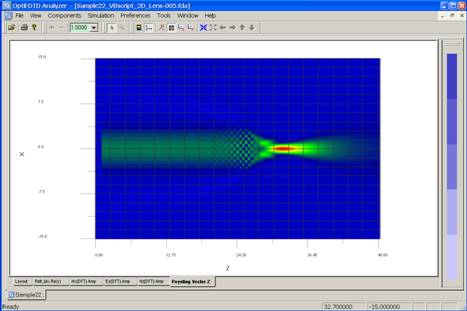Figure 2: Poynting vector for Fiber lens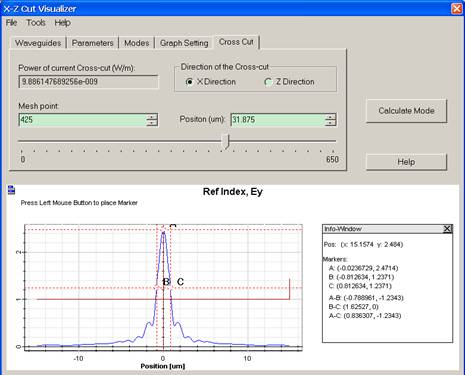Figure 3: Beam size measurement in OptiFDTD

Nano Lens Array

In this 3D sample, we discuss a micro-lens array, the lens dimension is marked in Figure 1, and Figure 2 is xy plane refractive index distribution.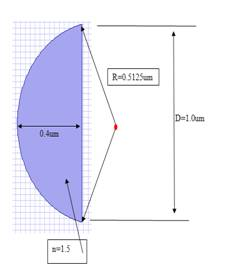Figure 1: Lens size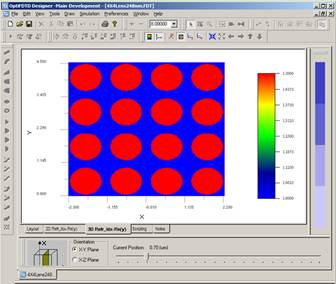Figure 2: Top view of the lens Array

Simulation Parameters

• • Mesh size : 0.02um*0.02um*0.02um
• • Run for 1248 time step +
• • Calculate steady state field for Ey and Hx (Discretized Fourier transform)
• • x-y boundary use the upper-lower symmetric boundary condition. Z-direction user the APML as the boundary condition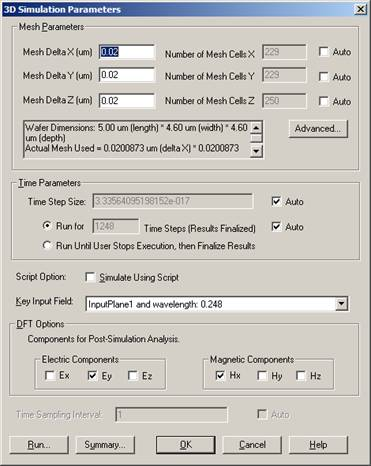Figure 3: Simulation parameters

Simulation results

As shown in figure 4, in the simulation we can observe the time domain response in each slice in three orientations ( xy plane, yz plane, xz plane)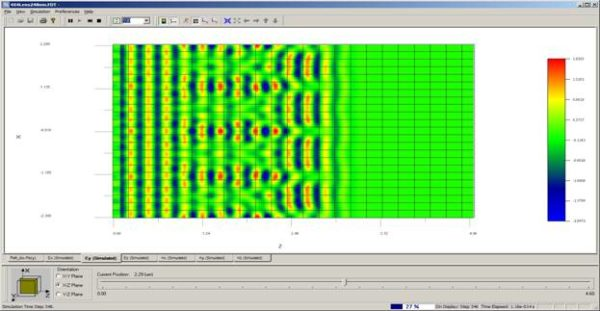Figure 4: Time domain response in xz plane

Steady state response can be observed in analyzer as shown figure 5. Beam size and focus distance can be measured in OptiFDTD as shown in figure 6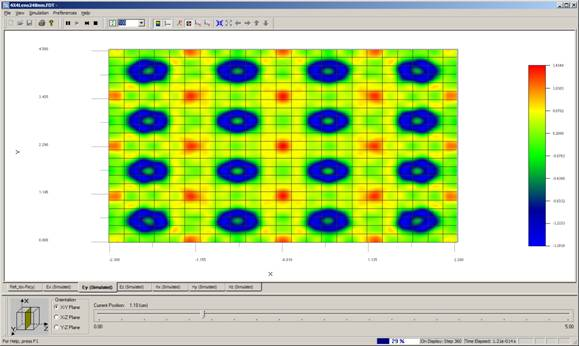Figure 5: Field pattern in xy plane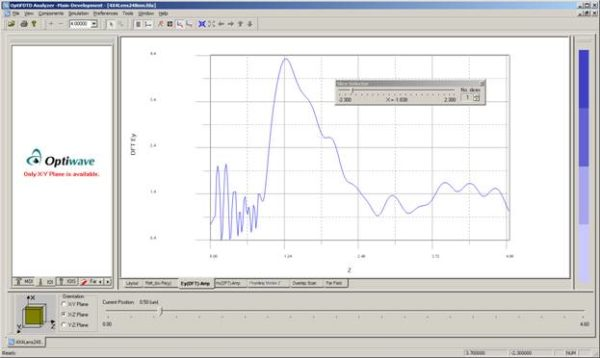Figure 6: Field pattern in xz plane

The calculation shows that each lens focuses at z=1.26, focus distance 1.26-0.8=0.46um